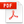2023

2022

Full text!

2021

Vol. 26 N° 6Vol. 26 N° 5Vol. 26 N° 4Vol. 26 N° 3Vol. 26 N° 2Vol. 26 N° 12020

Vol. 25 N° 6Vol. 25 N° 5Vol. 25 N° 4Vol. 25 N° 3Vol. 25 N° 2Vol. 25 N° 12019

Vol. 24 N° 6Vol. 24 N° 5Vol. 24 N° 4Vol. 24 N° 3Vol. 24 N° 2Vol. 24 N° 12018

Vol. 23 N° 6Vol. 23 N° 5Vol. 23 N° 4Vol. 23 N° 3Vol. 23 N° 2Vol. 23 N° 12017

Vol. 22 N° 10Vol. 22 N° 9Vol. 22 N° 8Vol. 22 N° 7Vol. 22 N° 6Vol. 22 N° 5Vol. 22 N° 4Vol. 22 N° 3Vol. 22 N° 2Vol. 22 N° 12016

Vol. 21 N° 10Vol. 21 N° 9Vol. 21 N° 8Vol. 21 N° 7Vol. 21 N° 6Vol. 21 N° 5Vol. 21 N° 4Vol. 21 N° 3Vol. 21 N° 2Vol. 21 N° 12015

Vol. 20 N° 10Vol. 20 N° 9Vol. 20 N° 8Vol. 20 N° 7Vol. 20 N° 6
Vol. 20 N° 5
Vol. 20 N° 4
Vol. 20 N° 3
Vol. 20 N° 2
Vol. 20 N° 1

2014

2013

2012

2011

2010

2009

2008

2007

2006

2005

2004

2003

2002

2001

2000

1999

1998

1997

1996